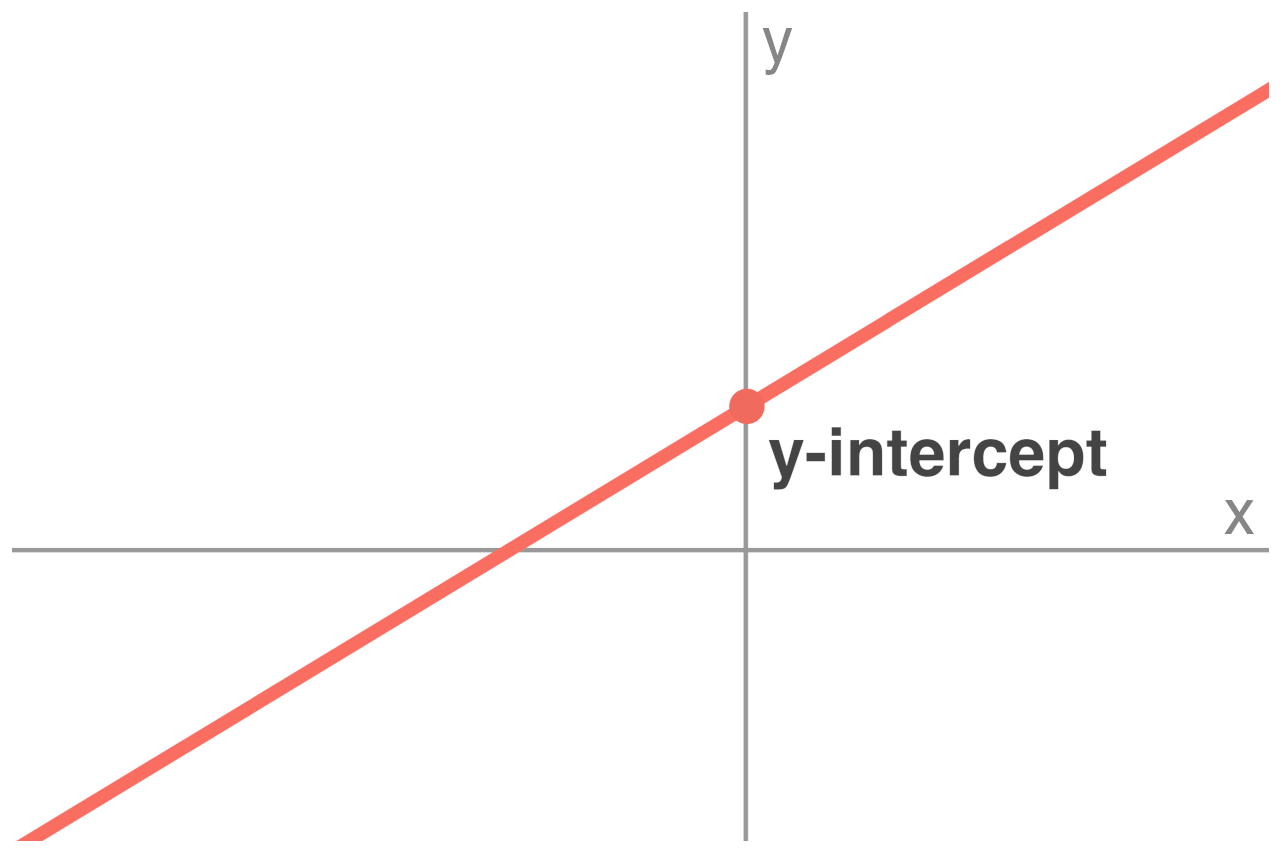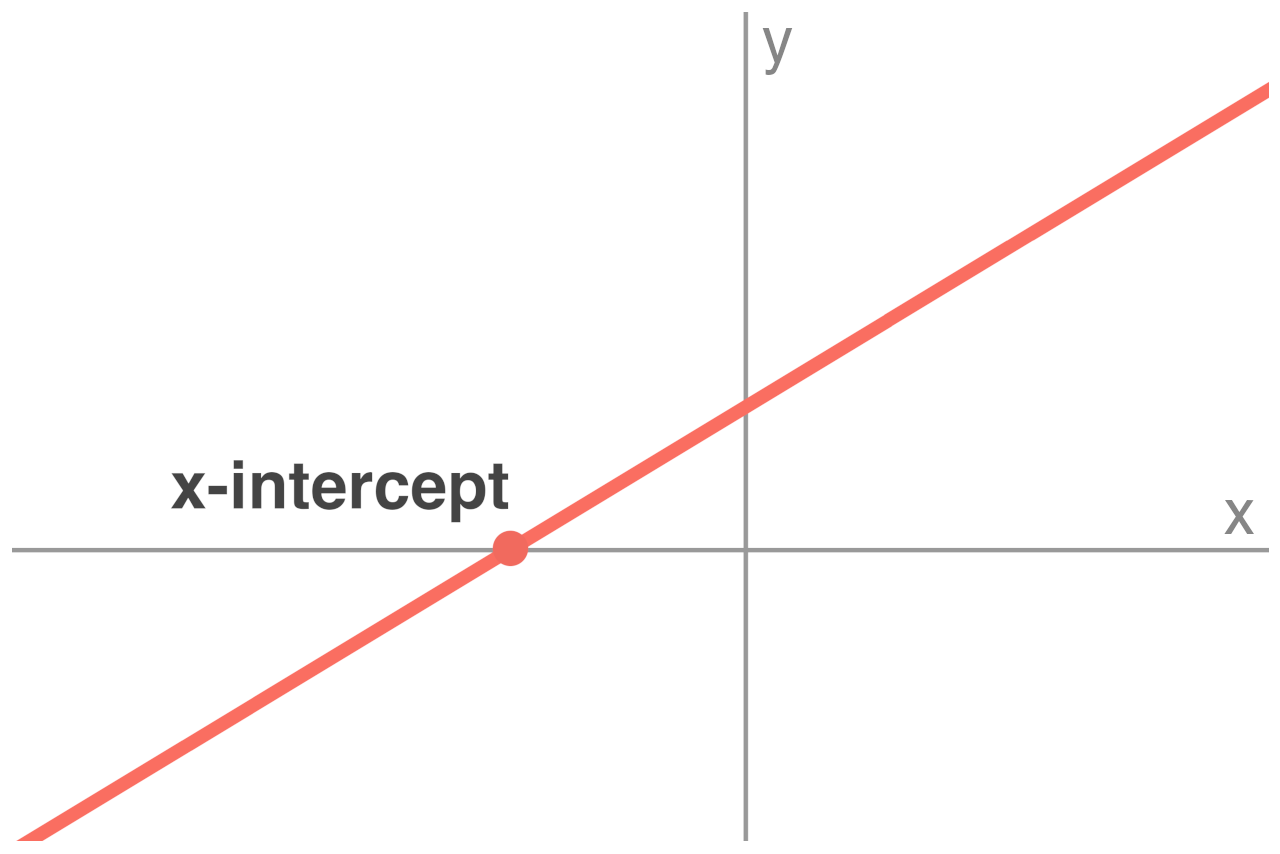X and Y Intercept Calculator

Find the x and y intercepts of a line using the intercept calculator below.

## X and Y Intercepts:

 x-intercept: -1 y-intercept: 1 slope (m): 1

### Line Graph:

 x-intercept: y-intercept: slope (m):

### Line Graph:

 x-intercept: y-intercept: slope (m):

### Line Graph:

 x-intercept: y-intercept: slope (m):

### Line Graph:

 x-intercept: y-intercept: slope (m):

### Line Graph:

• Calculator
• How to Find the Y-Intercept
• Y-Intercept of a Line in Slope-Intercept Form
• Y-Intercept of a Line in Standard Form
• Y-Intercept of a Line in Point-Slope Form
• How to Find the X-Intercept
• X-Intercept of a Line in Slope-Intercept Form
• X-Intercept of a Line in Standard Form
• X-Intercept of a Line in Point-Slope Form
• How to Find the Slope From the X and Y Intercept
• How to Graph a Line Using the Intercepts
• References

## How to Find the Y-Intercept

The y-intercept of a line or curve is the point where the line crosses the y-axis. More specifically, the y-intercept is the y-coordinate of the point on the line that has an x-coordinate equal to 0.Given the equation of a line, you can find the y-intercept by setting the value of the x variable in the equation equal to zero (0).

For example, given a linear function f(x) = y, you can find the y-intercept by setting x to 0 so that f(0) = b. For a line, you can use the following methods to calculate the point where the line crosses the y-axis.

### Y-Intercept of a Line in Slope-Intercept Form

Slope-intercept form is one of the most frequently used equations to express a straight line.

The slope-intercept form equation is:

y = mx + b

In the slope-intercept formula, the variable b is equal to the y-intercept. So the y-intercept is equal to b, or the point (0, b).

### Y-Intercept of a Line in Standard Form

Standard form (or general form) is the standard format for the equation of a straight line.

The standard form line equation is:

Ax + By = C

To find the y-intercept for a line in standard form, set the value of x to 0 and solve the equation for y.

A(0) + By = C
By = C
By / B = C / B
y = C / B

So, the y-intercept of the line is equal to C/B or the point (0, C/B).

Some uses of the standard form use an alternate version of the equation:

Ax + By + C = 0

In this variation of the equation, the y-intercept is slightly different:

A(0) + By + C = 0
By + C = 0
By + C – C = -C
By / B = –C / B
y = –C / B

### Y-Intercept of a Line in Point-Slope Form

Point-slope form is another commonly used linear equation format.

The point-slope form equation is given by:

y – y1 = m(x – x1)

Like before, set the value of x to 0 to solve for y.

y – y1 = m(0 – x1)
y – y1 = -mx1
y – y1 + y1 = -mx1 + y1
y = y1 – mx1

The y-intercept for a line expressed in point-slope form is equal to y1 – mx1 or the point (0, y1 – mx1).

## How to Find the X-Intercept

The x-intercept of a line or curve is the point where the line crosses the x-axis. The x-intercept is the x-coordinate of the point on the line that has a y-coordinate equal to 0.You can find the x-intercept of a line by following the same steps as solving the y-intercept, except that instead of setting the value of x to zero, you’ll be setting the value of y to 0.

### X-Intercept of a Line in Slope-Intercept Form

Recall the slope-intercept form equation from above:

y = mx + b

To find the x-intercept, set the value of y to 0 and solve for x.

0 = mx + b
0 – b = mx + b -b
-b = mx
–b / m = mx / m
–b / m = x

For a line in slope-intercept form, the x-intercept is equal to -b/m, or the point (-b/m, 0).

### X-Intercept of a Line in Standard Form

Recall the standard form equation from above:

Ax + By = C

To find the x-intercept, set the value of y to 0 and solve for x.

Ax + B(0) = C
Ax = C
Ax / A = C / A
x = C / A

For a line in standard form, the x-intercept is equal to C/A, or the point (C/A, 0).

### X-Intercept of a Line in Point-Slope Form

Recall the point-slope form equation is:

y – y1 = m(x – x1)

To solve the x-intercept, set the value of y to 0 and solve for x.

0 – y1 = m(x – x1)
-y1 = m(x – x1)
-y1 / m = m(x – x1) / m
-y1 / m + x1 = x – x1 + x1
x1 – y1 / m = x
-y1 + mx1 = mx – mx1 + mx1
-y1 + mx1 = mx
(-y1 + mx1) / m = mx / m
(mx1 – y) / m = x

Thus, the x-intercept for a line in point-slope form is (x1 – y1)/m, or the point (x1 – y1)/m, 0).

How to Find the Slope From the X and Y Intercept

Once you have the x and y intercepts for the line, you can find the slope of the line.

You’ll need the slope formula to find the slope:

m = y2 – y1 / x2 – x1

The intercepts can be treated just as any other points on the line. So, if you substitute the points (0, y) and (x, 0) into the slope formula, you can find the slope.

m = 0 – y / x – 0
m = –y / x

Thus, the slope of the line is equal to the negative y-intercept divided by the x-intercept.

## How to Graph a Line Using the Intercepts

Since the x-intercept and y-intercept are points on the line like any other, you can graph the line using them as well.

Plot the intercept points on the axes of the graph, the x-intercept being on the x-axis and the y-intercept being on the y-axis, then connect them with a line, extending the line indefinitely to both sides of the graph.

## References

You are watching: X and Y Intercept Calculator. Info created by GBee English Center selection and synthesis along with other related topics.